Common Core: 2nd Grade Math : Number & Operations in Base Ten

Example Questions

← Previous 1 3 4 5 6 7 8 9 114 115

Example Question #1 : Number & Operations In Base Ten

What digit is in the hundreds place?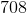Explanation:

The hundreds place is always the third number over from the right. In this case,is the third number over from the right.

Example Question #2 : Number & Operations In Base Ten

What digit is in the tens place?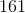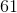Explanation:

The tens place is always the second number over from the right. In this case,is the second number over from the right.

Example Question #3 : Number & Operations In Base Ten

What digit is in the ones place?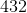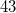Explanation:

The ones place is always the first number on the right. In this case,is the first number on the right.

Example Question #4 : Number & Operations In Base Ten

What digit in the hundreds place?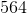Explanation:

The hundreds place is always the third number over from the right. In this case,is the third number over from the right.

Example Question #5 : Number & Operations In Base Ten

What digit is in the tens place?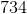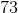Explanation:

The tens place is always the second number over from the right. In this case,is the second number over from the right.

Example Question #6 : Number & Operations In Base Ten

What digit is in the ones place?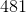Explanation:

The ones place is always the first number on the right. In this case,is the first number on the right.

Example Question #7 : Number & Operations In Base Ten

What digit is in the hundreds place?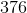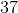Explanation:

The hundreds place is always the third number over from the right. In this case,is the third number over from the right.

Example Question #8 : Number & Operations In Base Ten

What digit is in the tens place?Explanation:

The tens place is always the second number over from the right. In this case,is the second number over from the right.

Example Question #9 : Number & Operations In Base Ten

What digit is in the ones place?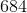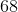Explanation:

The ones place is always the first number on the right. In this case,is the first number on the right.

Example Question #10 : Number & Operations In Base Ten

What digit is in the hundreds place?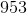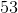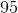The hundreds place is always the third number over from the right. In this case,is the third number over from the right.